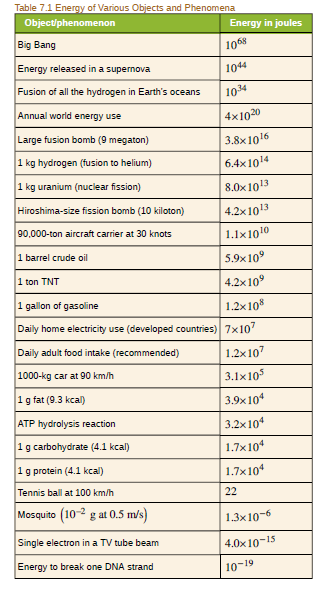Chapter 28, Problem 63PE

Chapter
Section
Textbook Problem

(a) Using data from Table 7.1, find the mass destroyed when the energy in a barrel of crude oil is released. (b) Given these barrels contain 200 liters and assuming the density of crude oil is 750 kg/m3, what is the ratio of mass destroyed to original mass, Δ m / m ?To determine

(a)

The mass destroyed.

Explanation

Given data:

The energy is E=5.90×109J.

The velocity of light is c=3.00×108m/s.

Formula used:

The relativistic kinetic energy is,

E=Δmc2

Substitute the given value.

Δm=5.90×109J(3

To determine

(b)

The ratio of mass destroyed and original mass.

Still sussing out bartleby?

Check out a sample textbook solution.

See a sample solution

The Solution to Your Study Problems

Bartleby provides explanations to thousands of textbook problems written by our experts, many with advanced degrees!

Get Started

Find more solutions based on key concepts